The florist

The florist had 200 roses in the morning. During the day, more than half sold it. From the remaining roses, it will tie the bouquet. If a bouquet of 3, 4, 5 or 6 roses are bound, one always remains. How many roses from the morning shipment sold?

Result

x =  139

Solution:Leave us a comment of this math problem and its solution (i.e. if it is still somewhat unclear...):Be the first to comment!To solve this verbal math problem are needed these knowledge from mathematics:

Do you want to calculate least common multiple two or more numbers?

Next similar math problems:

1. Three buses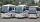Three public transport buses depart together from the bus station in the morning. The first bus was returning to the station after 18 minutes, the second after 12 minutes and a third after 24 minutes. How long will again together on the station? Result exp
2. 3 buses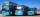At morning 5:00 am three buses started from one place. The first travel in five-minute intervals, the second at 10-minute intervals and the third at 25-minute intervals. At what hour will again be the three buses coming from the same place?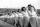Practitioners lined up in rectangle with row with four, five or six exercisers, one always missing to full rectangle. How many exercisers were on the field, if they have estimated not been more than 100?
4. TissuesThe store got three kinds of tissues - 132 children, 156 women and 204 men. Tissues each species were packed into boxes after the number of pieces the same for all three types (and greatest). Determine the number, if you know that every box has more than 6
5. Digit sumDetermine for how many integers greater than 900 and less than 1,001 has digit sum digit of the digit sum number 1.
6. Tailor master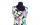There are less than 50m of textile in the tailoring workshop. When cutting on a blouse (consumption 1.5m), no textile is left. When using a cloth (consumption of 3.2m), no textile is left. How many meters of textile are in a tailor's workshop?
7. Two-digit numberI am a two-digit number less than 20. When I divided by three, then you get the rest 1 and when you divide me by four you get also rest 1. What number am I?
8. Trams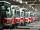Tram no. 3,7,10,11 rode together from the depot at 5am. Tram No. 3 returns after 2 hours, tram No. 7 an hour and half, no. 10 in 45 minutes and no. 11 in 30 minutes. For how many minutes and when these trams meet again?
9. Class 9AOn the final certificate have one quarter of the class 9A mark "C" of mathematics, seventh mark "C" from the Czech language and two students failed in chemistry. How many students attend class 9A?
10. Dance groupThe dance group formed groups of 4, 5, and 6 members. Always one dancer remains. How many dancers were there in the whole group?
11. Bouquet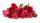Gardener tying bouquet of flowers for 8 and none was left. Then he found that he could tying bouquet of 6 flowers and also none was left. How many have gardener flowers (minimum and maximum) if they had between 50 and 100 flowers?
12. Divisibility by 12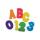Replace the letters A and B by digits so that the resulting number x is divisible by twelve /find all options/. x = 2A3B How many are the overall solutions?
13. RemainderA is an arbitrary integer that gives remainder 1 in the division with 6. B is an arbitrary integer that gives remainder 2 the division by. What makes remainder in division by 3 product of numbers A x B ?
14. Matches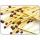George poured out of the box matches and composing them triangles and no match was left. Then he tries squares, hexagons and octagons and no match was left. How many matches must be at least in the box?
15. Six-digit primesFind all six-digit prime numbers that contain each one of digits 1,2,4,5,7 and 8 just once. How many are they?
16. Toy carsPavel has a collection of toy cars. He wanted to regroup them. But in the division of three, four, six, and eight, he was always one left. Only when he formed groups of seven, he divided everyone. How many toy cars have in the collection?
17. LCM of two numberFind the smallest multiple of 63 and 147